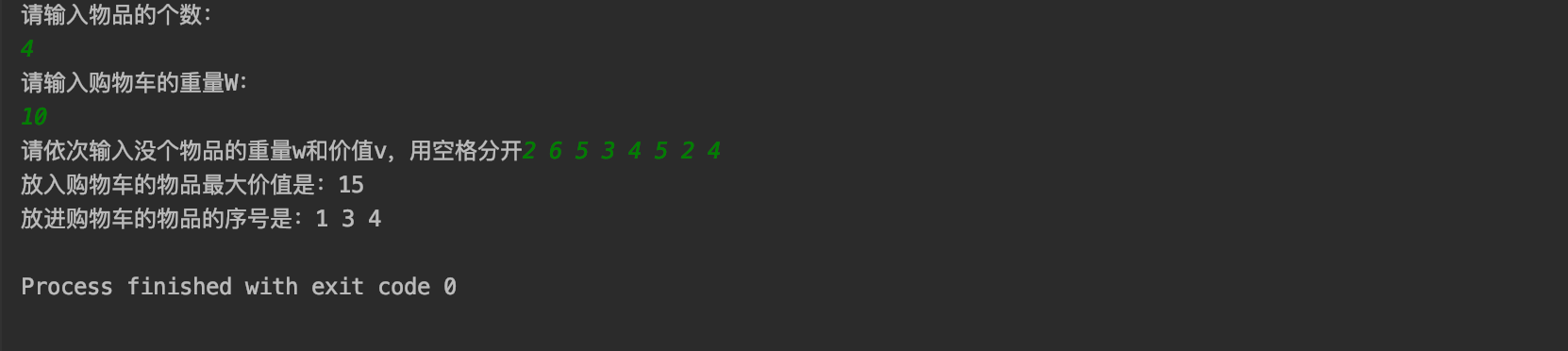01背包典型的解法是动态规划，之前的博客也有介绍，这里就不再赘述。

https://blog.csdn.net/Jayphone17/article/details/102553763

https://blog.csdn.net/Jayphone17/article/details/102910824

（1）定义问题解空间

（2）确定解空间组织结构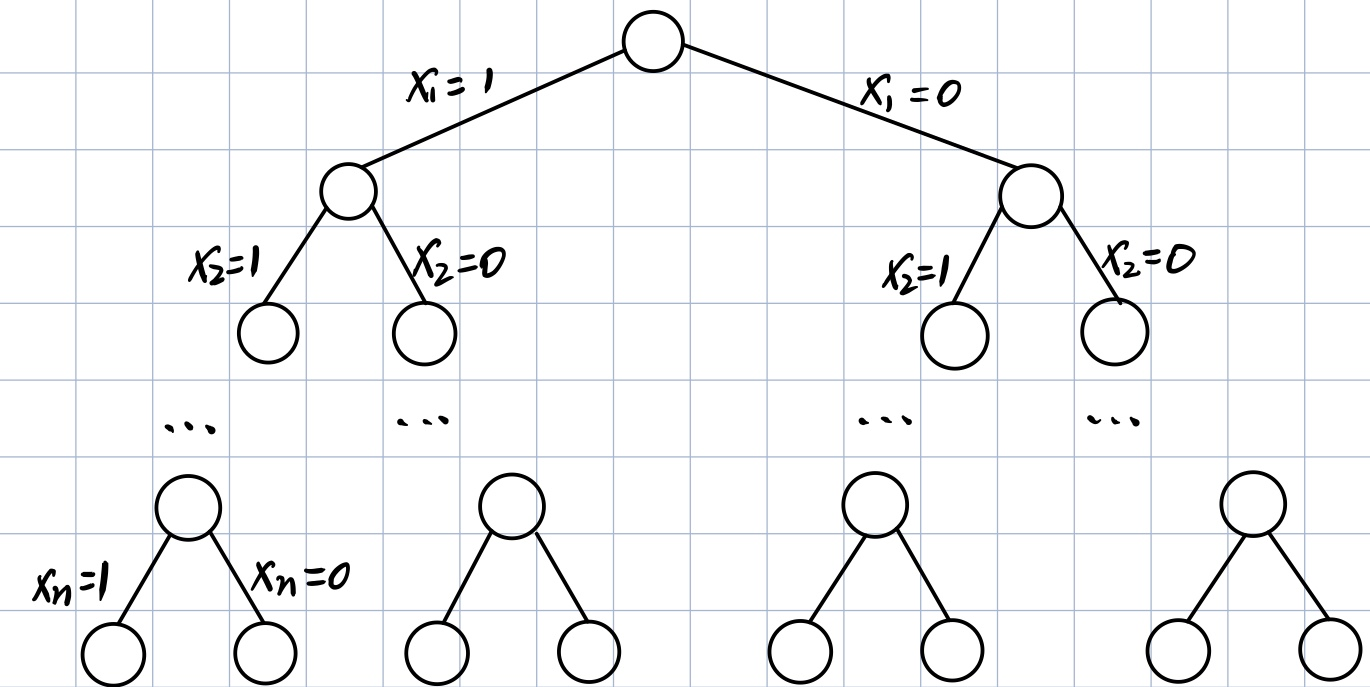（3）搜索解空间树

1.约束条件：

2.限界条件：

• 如果价值上界小于或者等于当前搜索到的最优值（用bestp表示，初始值为0），则说明从中间结点z继续向子孙结点搜索不可能得到一个更优解，没有搜索的必要（剪枝），反之，继续向子孙结点搜索。
• 限界条件为：cp+rp<=bestp

3.搜索过程：

• 如果成立，则扩展当前结点生成左子树，继续进行深度优秀搜索，如此往复。
• 如果不成立，剪掉扩展结点的左分支，对右分支进行扩展，代表不装进购物车。在此时，沿着右子树也可能生成最优解，所以我们这时候要判断 限界条件 ，如果满足，则说明有可能生成最优解，此时右结点成为活结点，继续扩展。
• 如果不满足 限界条件 剪掉扩展的右边分支，向最近的 父结点 回溯。
• 直到死结点。

（1）初始化。

sumw=13，sumv=18，sumw>10，说明不可以全部装完，需要搜索求解。

（2）扩展根结点，生成二号结点（第一层）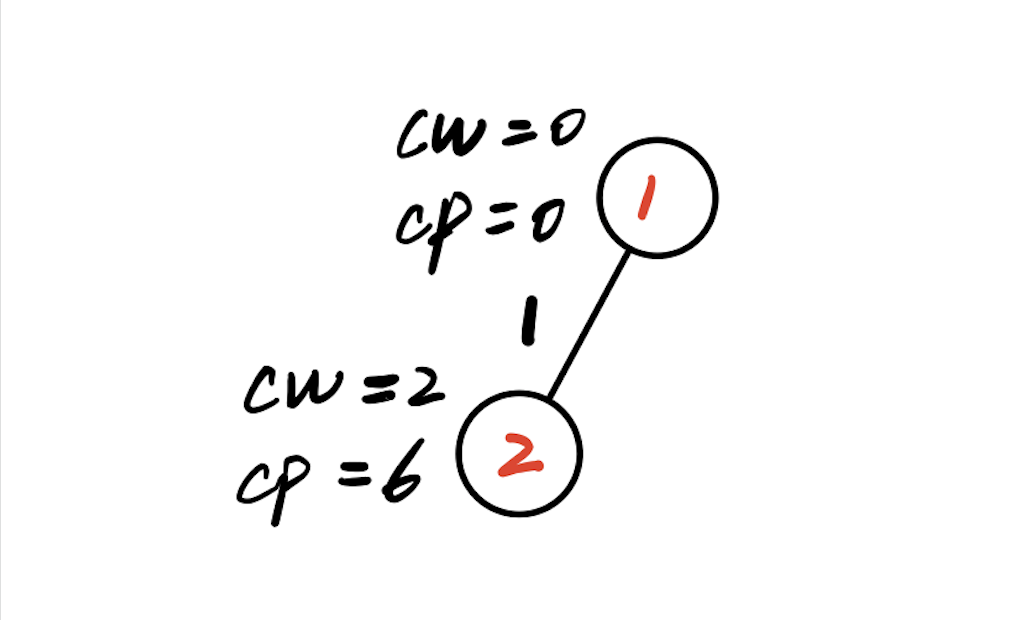（3）此时重量cw<10，继续扩展二号结点（第二层）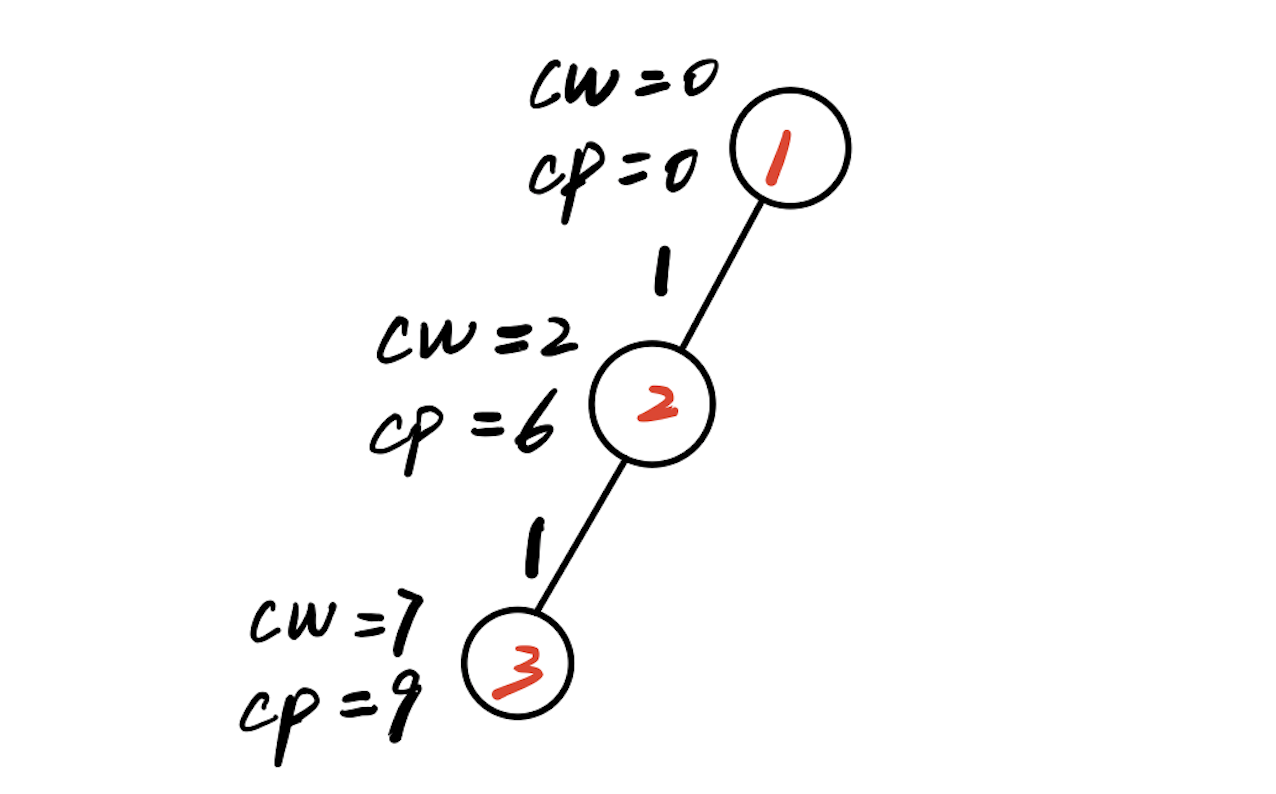（4）此时cw+w=11>W=10，说明第三个物品不能犯禁，那么判断cw+cp是否大于bestp，此时rp=4，cp+rp=13，bestp=0，因此满足限界条件，扩展右子树，生成4号结点（第三层）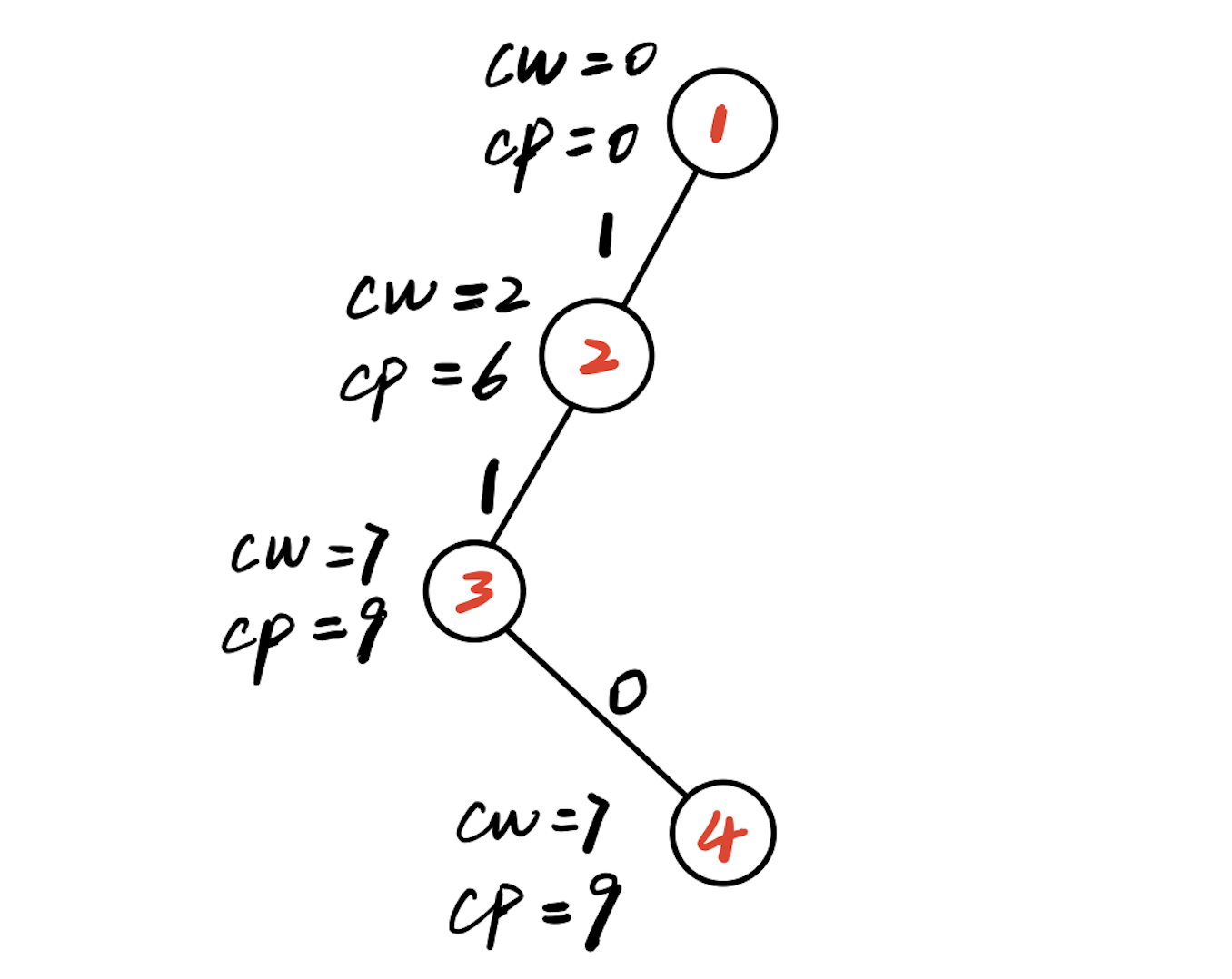（5）扩展4号结点，首先判断cw+w=9<10=W，满足约束条件，扩展左分支，生成5号结点（第四层）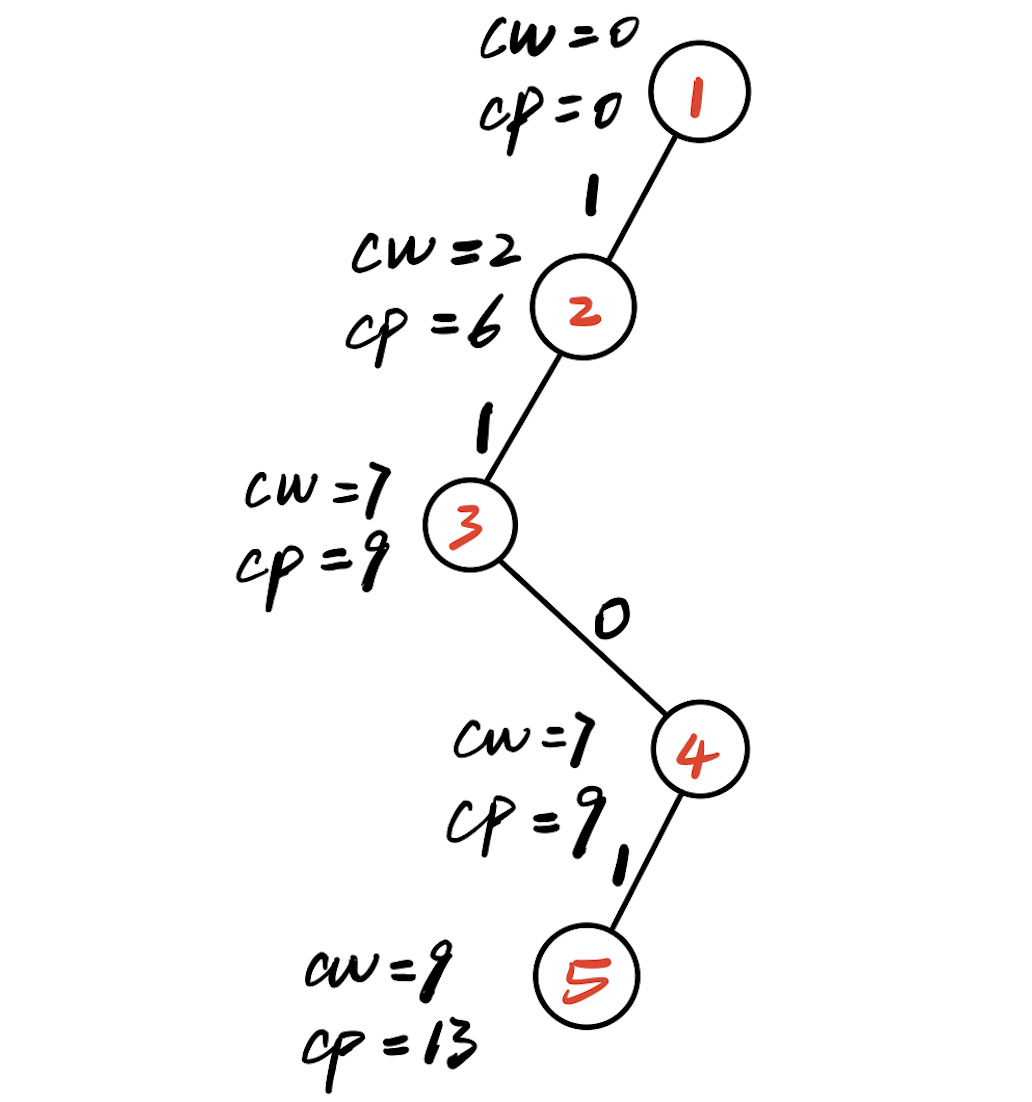（6）此时5号结点的深度是5>n=物品的个数，此时找到一个最优解，更新最优解的值bestp=13。此时5号结点已经成为死结点。搜索结束，开始回溯。

（7）回溯到4号结点，此时没有剩余物品，rp=0，cp=9，cp+rp=9<13，因此不扩展右边结点，剪枝，所以4号结点成为死结点。

（8）回溯到3号结点，3号结点的左分支已经剪枝，所以3结点也成为死结点。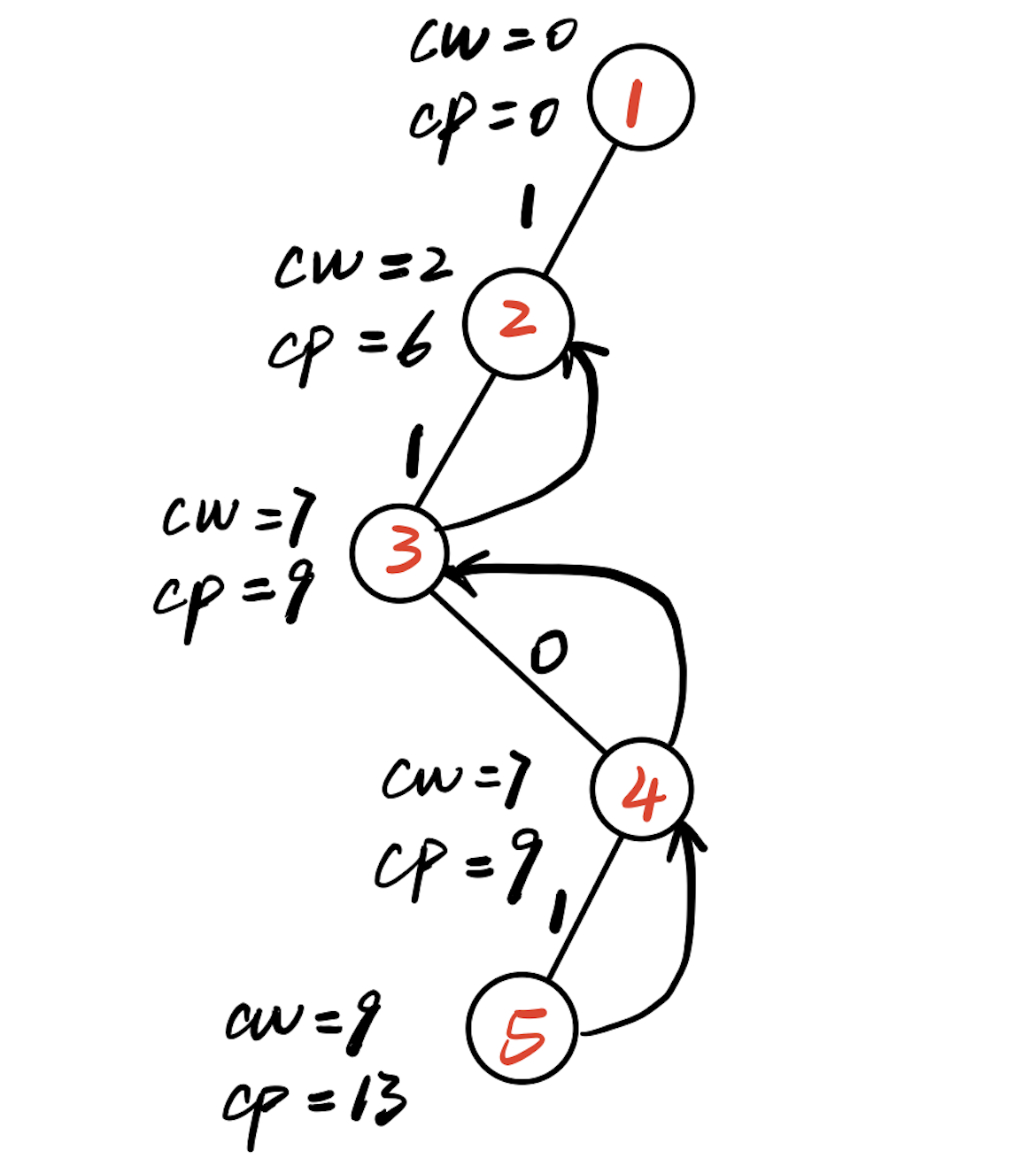（9）回溯到2号结点，此时剩余物品有两个，rp=9，cp=6，cp+rp=15>13=bestp，故扩展右分支，生成6号结点。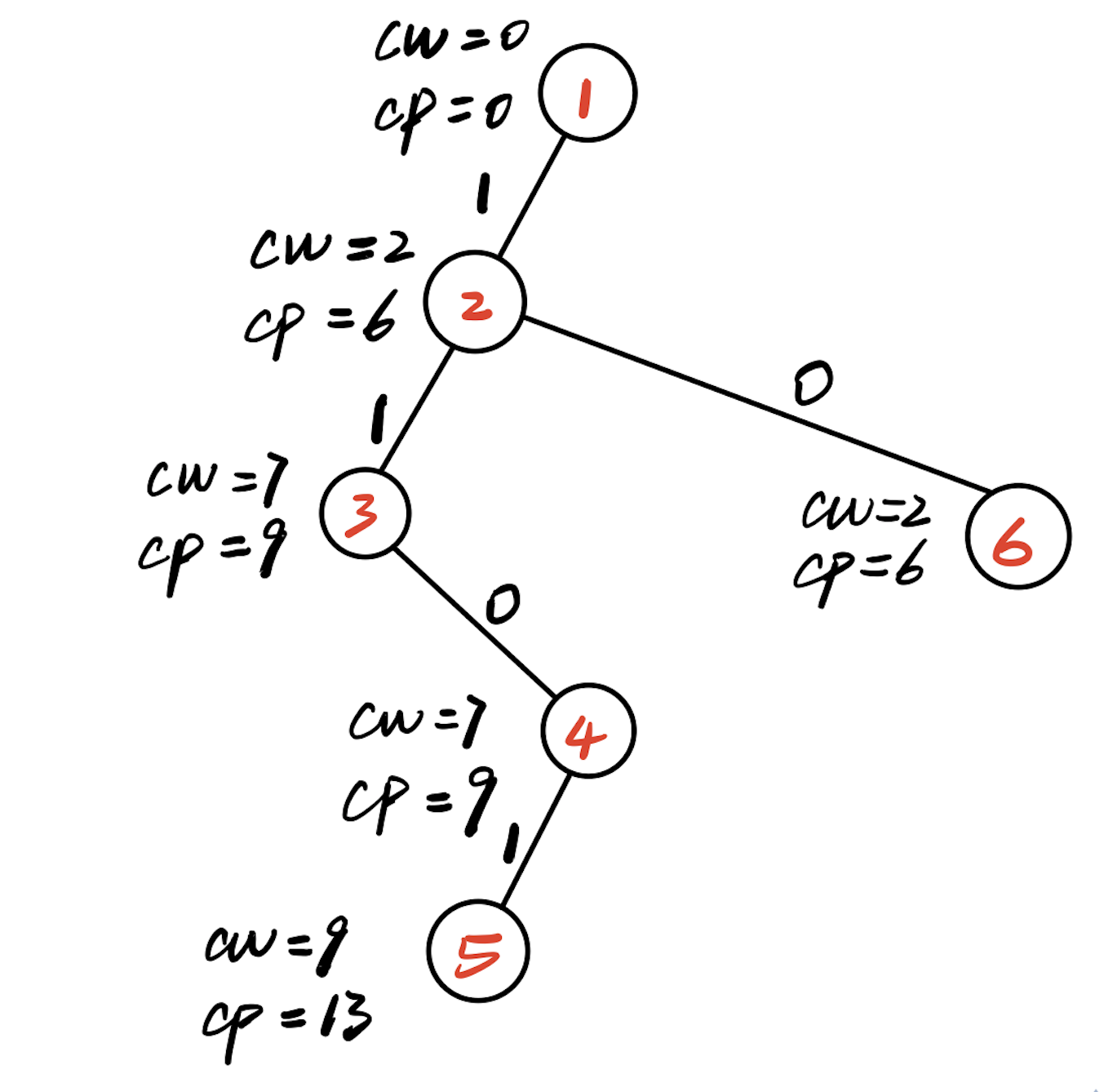（10）判断6号结点，cw+w=6<W，满足约束条件，扩展左分支，生成7号结点。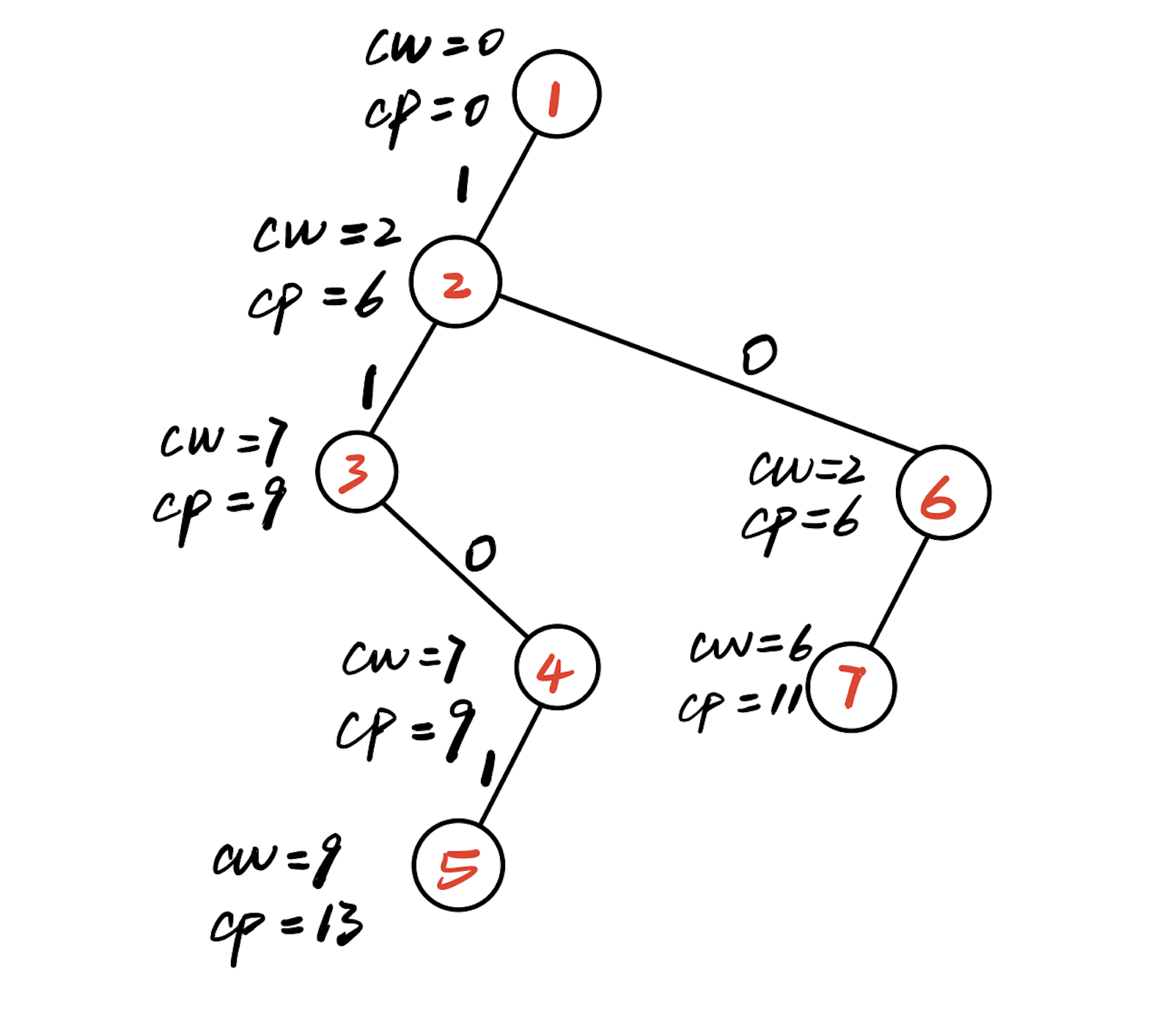（11）判断7号结点，cw+w=8<W，满足约束条件，扩展左分支，生成8号结点。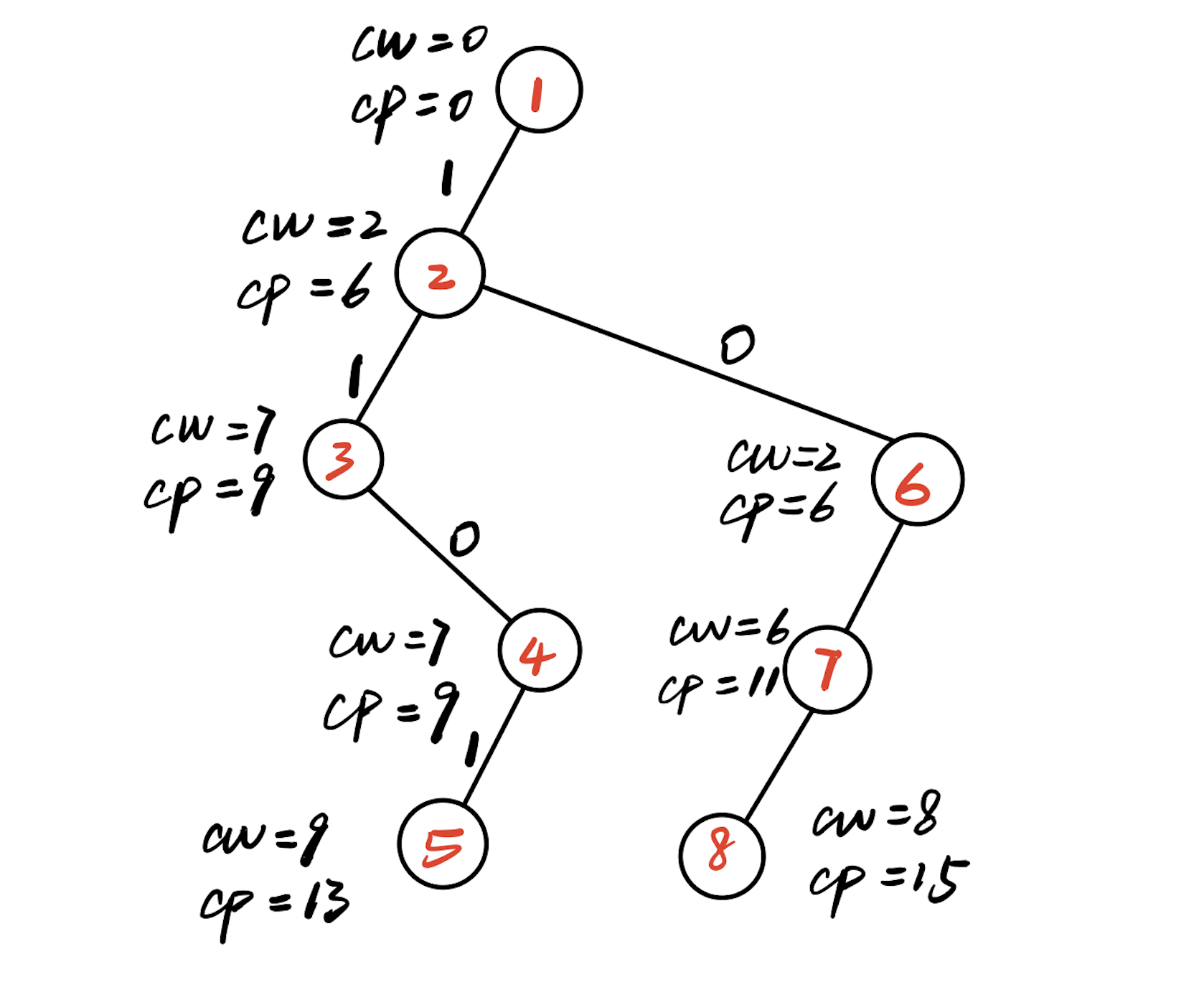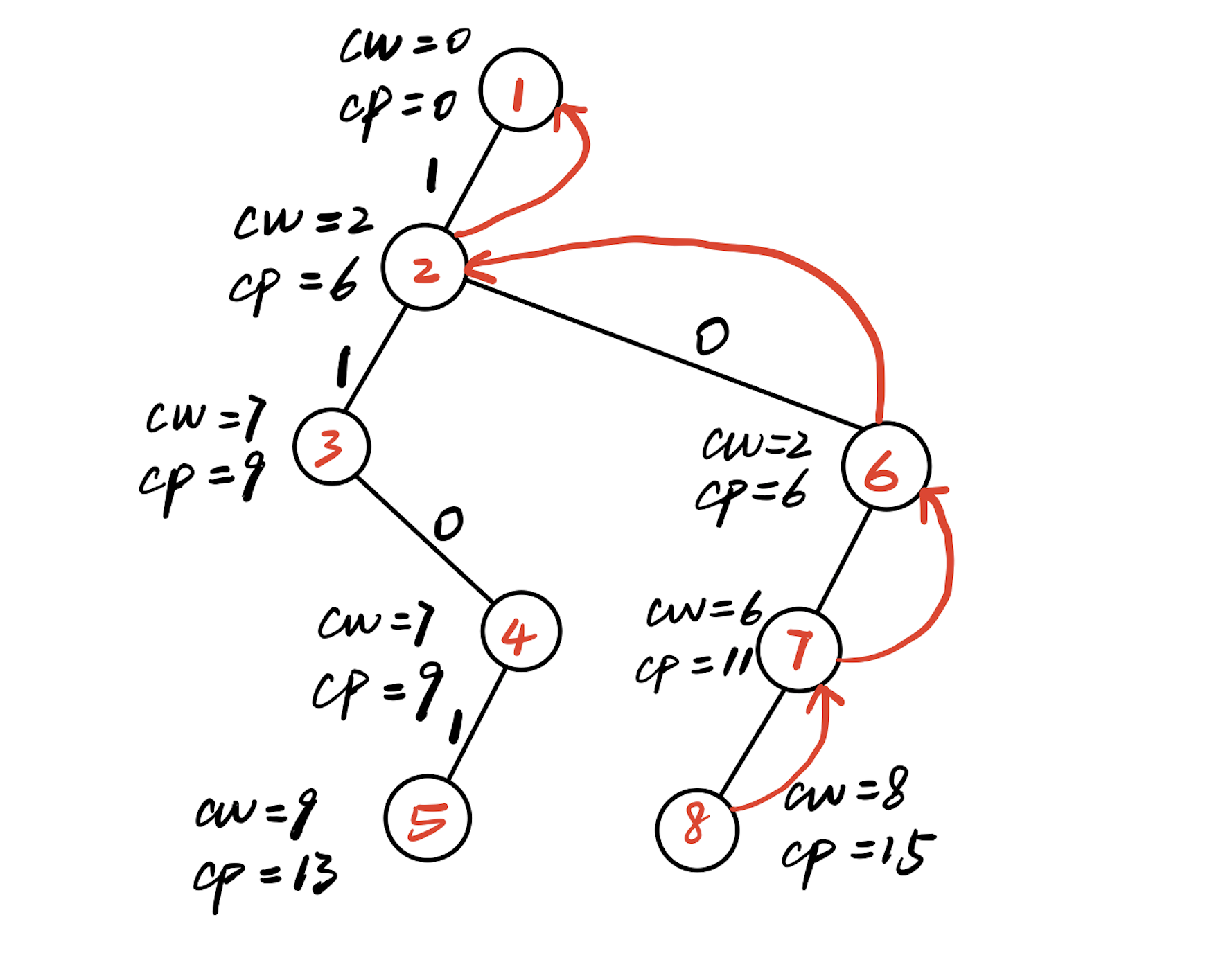（12）回溯到7号结点，此时没有剩余物品，rp=0，cp=11，cp+rp=11<bestp=15，不满足限界条件，不扩展7号结点的右子树。7号结点成为死结点。

（13）回溯到6号结点，此时剩余一个物品，rp=4，cp=10，rp+co=14<bestp=15，不满足限界条件，不扩展6号结点的右子树。6号结点成为死结点。

（14）回溯到2号结点，此时左右孩子都已经考察过，所以成为死结点。

（15）回溯到1号结点，此时剩余3个物品，rp=12，cp=0，rp+cp=12<bestp=15，不满足限界条件，所以不扩展1号结点的右子树，1号结点成为死结点。此时搜索结束。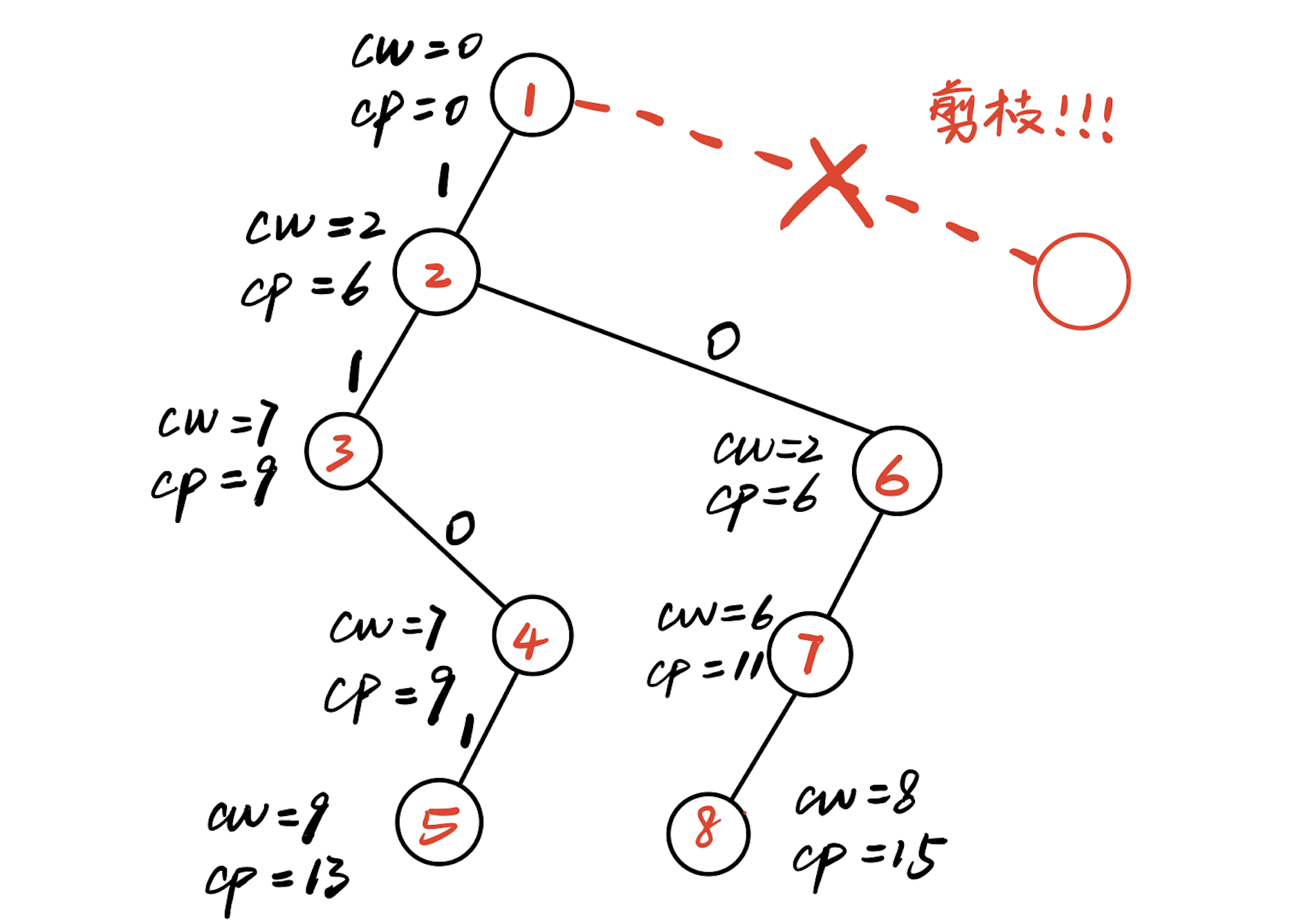（1）计算上界

double Bound(int i) //计算上界
{
int rp=0;
while(i<=n) //计算剩余物品重量和
{
rp+=v[i];
i++;
}
return cp+rp;
}


（2）按照约束条件和限界条件进行搜索

t表示当前扩展结点在t层，cw表示当前已经放进购物车的重量和，cp表示已经放进购物车的价值和。

void Backtrack(int t) //回溯函数
{
if(t>n)//已经到达了叶子结点
{
for (int j=1;j<=n;j++)
{
bestx[j]=x[j]; //记录编号
}
bestp=cp;//保留当前最优值
return;
}

if(cw+w[t]<=W)//如果满足条件，扩展并且搜索左子树
{
x[t]=1;
cw+=w[t];
cp+=v[t];
Backtrack(t+1);//回溯
cw-=w[t];
cp-=v[t];
}

if(Bound(t+1)>bestp)//如果满足限界条件，搜索右子树
{
x[t]=0;
Backtrack(t+1);//回溯
}
}

#include <iostream>
#include <algorithm>
#include <cstring>
using namespace std;
#define M 105

int n;//表示物品个数
int W;//表示购物车总载重量
double w[M];//表示对应物品重量
double v[M];//表示对应物品价值
bool x[M];//用来判断是否是否放入购物车
double cw;//当前放进购物车总重量
double cp;//当前放进购物车总价值
double bestp; //当前最优值
bool bestx[M]; //当前最优解

double Bound(int i) //计算上界
{
int rp=0;
while(i<=n) //计算剩余物品重量和
{
rp+=v[i];
i++;
}
return cp+rp;
}

void Backtrack(int t) //回溯函数
{
if(t>n)//已经到达了叶子结点
{
for (int j=1;j<=n;j++)
{
bestx[j]=x[j]; //记录编号
}
bestp=cp;//保留当前最优值
return;
}

if(cw+w[t]<=W)//如果满足条件，扩展并且搜索左子树
{
x[t]=1;
cw+=w[t];
cp+=v[t];
Backtrack(t+1);//回溯
cw-=w[t];
cp-=v[t];
}

if(Bound(t+1)>bestp)//如果满足限界条件，搜索右子树
{
x[t]=0;
Backtrack(t+1);//回溯
}
}

void Knapsack(double W,int n)
{
//初始化
cw=0;
cp=0;
bestp=0;
double sumw=0;
double sumv=0;
for (int i=1;i<=n;i++)
{
sumw+=w[i];
sumv+=v[i];
}
if(sumw<=W)
{
bestp=sumv;
cout << "放进购物车的物品最大价值是：" << bestp << endl;
return;
}
Backtrack(1);//回溯
cout << "放入购物车的物品最大价值是：" << bestp << endl;
cout << "放进购物车的物品的序号是：";
for (int i=1;i<=n;i++)
{
if(bestx[i]==1)
{
cout << i << " " ;
}
}
cout << endl;
}
int main()
{
cout << "请输入物品的个数："  << endl;
cin >>n;
cout << "请输入购物车的重量W：" << endl;
cin>>W;
cout << "请依次输入没个物品的重量w和价值v，用空格分开";
for (int i=1;i<=n;i++)
{
cin >> w[i] >> v[i];
}
Knapsack(W,n);
return 0;
}